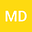•We considered an incompressible fluid-saturated porous layer bounded by two infinite parallel plates. Boussinesq approximation and Darcy’s law are applied. The permeability is assumed to be a linear function of the depth $z$. The linear stability is investigated. The long wavelength expansion method is applied to conduct the weakly nonlinear stability analysis. The evolution equation is derived and analyzed. A uniformly valid periodic solution of the evolution equation is obtained by the application of Poincar\’e-Lindstedt method. Some numerical simulations is presented.